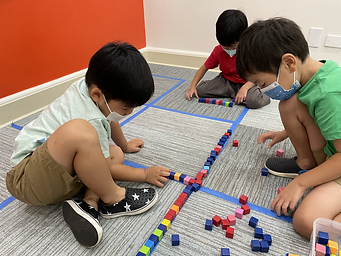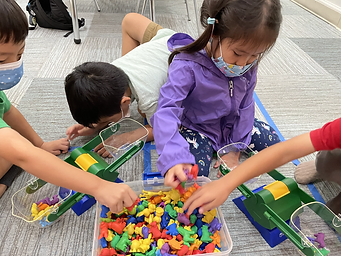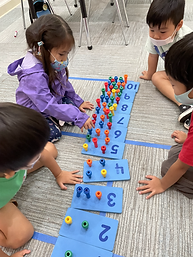## Ms. Kayla

### Target 1​

###### Lesson Type:

New

Measurment

:

Comparison

Visually distinguish between items based on measurable criteria.

###### 1:

Use a given reference unit to compare the length of objects.

###### 2:

Understand that the more units an object has the longer it is.

Pre-K

###### Vocabulary:

Measure, Length, Long

Activities:

1. Students were introduced to the idea of using a reference unit to measure length. Students measured their hand, feet, and carpet squares. Students made sure to not leave any gaps/spaces between their unit of measure when they were measuring an object.

2. Students were also introduced to a scale/balance to measure the weight of an object. Students discovered when a heavier object was put on the balance, it would go down and the other side would go up meaning the other object was lighter.### Home Exploration

###### Guiding Questions:## Absent Students:

### Target 2

:

###### 1:

Draw squares, rectangles, circles, and triangles.

Pre-K

###### Vocabulary:

Circle, Draw

Activities:

1. Students drew circles for the seeds of their strawberry.### Home Exploration

###### Guiding Questions:### Target 3

:

###### 1:

Use one-to-one correspondence when counting to connect one quantity with one number name.

###### 2:

Understand that each successive number in the counting sequence is one larger than the previous number.

###### 3:

Count objects to determine how many there are.

Pre-K

###### Vocabulary:

Count, Number, After, Before, Backwards

Activities:

1. Students used pegs to practice counting and identify numbers 1-10.

2. Students worked together to put the numbers in order.

3. Students practiced counting forward and backward.### Home Exploration